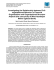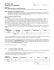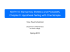# Chapter 9: Testing a Claim

## Transcription

Chapter 9: Testing a Claim
```The Practice of Statistics (4th Edition) - Yates, Moore, & Starnes
Chapter 9: Testing a Claim
Key Vocabulary:







Null Hypothesis
Alternative Hypotheses
Inference Toolbox
test statistic
p-value
 level
one-sample z statistic







statistically significant
Type I Error
Type II Error
power
standard error
t distribution
degrees of freedom






paired t-test
one-sample z statistic
one-sample t statistic
two-sample t statistic
robust
power
9.1 Significance Tests: The Basics (pp.528-546)
1. What is a significance test?
2. What is a null hypothesis?
3. What is an alternative hypothesis?
4. Explain the difference between a one-sided alternative hypothesis and a two-sided alternative
hypothesis.
5. In statistics, what is meant by the P-value?
6. If a P-value is small, what do we conclude about the null hypothesis?
7. If a P-value is large, what do we conclude about the null hypothesis?
8. If you fail to reject Ho, can you accept it?
Chapter 9: Testing a Claim
The Practice of Statistics (4th Edition) - Yates, Moore, & Starnes
9. What is meant by a significance level?
10. What does it mean if we say this is statistically significant?
11. On what evidence would we reject the null hypothesis?
12. On what evidence would we accept the null hypothesis (ie. fail to reject the null hypothesis)?
13. What is a Type I Error?
14. What is a Type II Error ?
15. What is the relationship between the significance level  and the probability of Type I Error?
16. What is meant by the power of a significance test?
17. State two ways to increase the power of a significance test:


Chapter 9: Testing a Claim
The Practice of Statistics (4th Edition) - Yates, Moore, & Starnes
9.2
Tests about a Population Proportion (pp.549-562)
1. What conditions must be verified before carring out a significance test for a population
proportion?
2. State the general form of the “test statistic”.
3. What does the test statistic measure?
4. What 4 steps should you follow when you are prefroming a significance test?




5. State the null hypothesis for a one-sample z test for a proportion.
6. Give the formula for the one-sample z test for a proportion, and define each variable in the
equation.
7. State and use diagrams to illustrate the three possible alternative hypotheses for a one
proportion z-test.
Chapter 9: Testing a Claim
The Practice of Statistics (4th Edition) - Yates, Moore, & Starnes
8. If no α level is given what should you use?
9. What additional information does a confidence interval provide that a significance test does
not?
9.3
Tests about a Population Mean (pp.565-587)
1. State the null hypothesis for a one sample t-test.
2. State and use diagrams to illustrate the three possible alternative hypotheses for a one sample
t-test
3. Give the formula for the one-sample t-statistic, and define each variable in the equation.
4. When can we use one-sample t-test?
5. When should a paired t- test be used, and what would be the statistic of interest?
Chapter 9: Testing a Claim
```

### AP Statistics – Chapter 9 Notes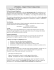### Population vs. Sample - Ms. Tomas` Math Page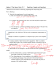### File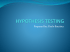### Chapter 11 and 12 Review 2015 ANSWERS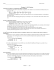### chapter 9 practice - faculty.piercecollege.edu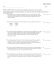### What is Hypothesis Testing? AP Statistics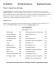### Ch 9 Lesson 1 Introduction Tests of Significance.jnt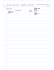### Math 119 Sample Final Exam Open book and note Calculator OK Multiple Choice  1 point each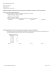### 155S8.5_3 Testing a Claim About a Mean: s Not Known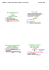### DTC Quantitative Methods Statistical Inference II: Statistical Testing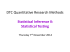### Using Your TI-NSpire Calculator for Hypothesis Testing: t Dr. Laura Schultz Statistics I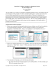### Inferential Statistics t and ScWk 242 – Session 9 Slides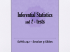### Chapter 9: Testing a Claim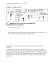### Sections 8-1 and 8-2 - Gordon State College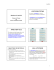### Notes to supplement Handout 11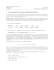### Review - Varsity Math by Coach G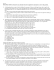### Chapter 7 Lecture Notes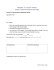### 1 Review: Four Steps to Construct a Confidence Interval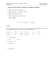### Full Text Pdf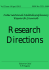### Investigating the Relationship between Civil